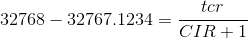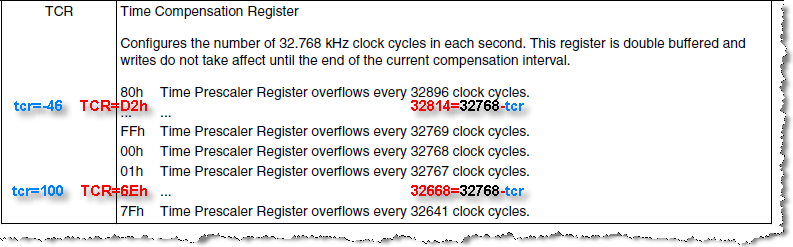How to Use the Kinetis RTC Time Compensation Register (RTC_TCR)?

cancel
Showing results for
Did you mean:
SOLVED

How to Use the Kinetis RTC Time Compensation Register (RTC_TCR)?

977 ViewsContributor IV

I read the AN4949 application note http://cache.freescale.com/files/microcontrollers/doc/app_note/AN4949.pdf

but it refers to Kinetis-M devices and I still don't understand how to set RTC_TCR register for frequency compensation in a Kinetis-K device like.

The CIR field set the compensation interval in seconds (from 1 to 256 s): every compensation interval the prescaler value is adjusted for one cycle with the value of the TCR field (+128 / -127) from the 32768 nominal value, right?

Now, I can measure the RTC_CLKOUT signal frequency, which quite always differs from the 32768 Hz nominal value; but how to calculate the right values for CIR and TCR fields?

If I measure RTC_CLKOUT = 32767.0000 Hz, should I set CIR = 0 and TCR = 1 ?

But what if I measure RTC_CLKOUT with a fractional part, i.e. 32767.1234 or 32768.8765 Hz ?

Regards

Labels (1)
• Kinetis K Series MCUs

1 Solution
563 ViewsNXP TechSupport

Hi Alessandro,

Please refer the equation shown below:tcr:   -127~128

CIR:   0~255

Best Regards,

Robin

-----------------------------------------------------------------------------------------------------------------------
-----------------------------------------------------------------------------------------------------------------------

5 Replies
564 ViewsNXP TechSupport

Hi Alessandro,

Please refer the equation shown below:tcr:   -127~128

CIR:   0~255

Best Regards,

Robin

-----------------------------------------------------------------------------------------------------------------------
-----------------------------------------------------------------------------------------------------------------------

563 ViewsContributor IV

Thank you, Robin.

So the right values for CIR and TCR fields are the one that in fractional mode best approximate the difference between the nominal value and the measured value of the RTC oscillator frequency, right?

Then I should embed the following procedure in my firmware application:

1. Calculate the difference between the nominal value and the measured value of the RTC oscillator frequency, i.e D = Fnom - Fmeasure
2. Convert D from signed decimal number in signed fractional number, i.e D = N / M
3. Calculate the equivalent minimum terms fractional number, i.e. D = n / m
4. Find the TCR value in the range [-127, 128] and CIR value in the range [0, 255] that best approximate D = n / m = TCR/(CIR + 1)

Is it right?

Or there is a better and faster way to get both TCR and CIR values?

Dealing with fractional approximations is not so easy to code....

563 ViewsContributor IV

Hi Robin,

From the formula above I can get the tcr and CIR values.

tcr may result positive or negative (signed char).

The TCR field in the RTC_TCR register should be set with the exact tcr value (with sign) or not?

For example:

32768 - 32769.846 = tcr / (CIR + 1)

I get:

tcr = -46 = 0xD2

CIR = 24

In the RTC_TCR register should I set TCR = 0xD2?

Another example:

32768 - 32767.123 = tcr / (CIR + 1)

I get:

tcr = 110 = 0x6E

CIR = 124

In the RTC_TCR register should I set TCR = 0x6E?

Best Regards

Alessandro

564 ViewsNXP TechSupport

Every (CIR+1)second apply (32768-tcr):For example:

32768 - 32769.846 = tcr / (CIR + 1)

I get:

tcr = -46 = 0xD2

CIR = 24

In the RTC_TCR register should I set TCR = 0xD2?

Yes

if every (CIR+1)second apply (32768-tcr)

if every (25)second apply (32814)

[32768*(CIR+1)-(tcr)]/32769.846Hz=[32768*(25)-(-46)]/32769.846Hz=24.999995422621149943762323448209sec

if not apply:

[32768*(25)]/32769.846Hz=24.998591693107132697541514232322sec

Another example:

32768 - 32767.123 = tcr / (CIR + 1)

I get:

tcr = 110 = 0x6E

CIR = 124

In the RTC_TCR register should I set TCR = 0x6E?

Yes

if every (CIR+1)second apply (32768-tcr)

if every (125)second apply (32668):

[32768*(CIR+1)-(tcr)]/32767.123Hz=[32768*(125)-(100)]/32767.123Hz=125.00029373955107380040658436812sec

if not apply:

[32768*(125)]/32767.123Hz=125.00334557904274964878668169921sec

Best Regards,

Robin

-----------------------------------------------------------------------------------------------------------------------
-----------------------------------------------------------------------------------------------------------------------

564 ViewsContributor IV

Hi Robin,

Can you confirm the following?

First case:

RTC_CLKOUT = 32769.846

32768 - RTC_CLKOUT = tcr / (CIR + 1)

I get:

tcr = -46

CIR = 24

The RTC is early, so every (CIR + 1) = 25 sec the RTC prescaler register should be adjusted in order to count (32768 + 46) = 32814 cycles for one second.

So TCR should be set to -46, i.e. TCR = 0xD2

Second case:

RTC_CLKOUT = 32767.123

32768 - RTC_CLKOUT = tcr / (CIR + 1)

I get:

tcr = 110

CIR = 124

The RTC is late, so every (CIR + 1) = 125 sec the RTC prescaler register should be adjusted in order to count (32768 - 110) = 32658 cycles for one second.

So TCR should be set to 110, i.e. TCR = 0x6E

Best Regards

Alessandro